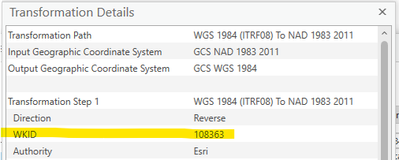# arcgis.geometry.project Not Returning Correct Coordinates

135
2
06-15-2022 11:52 AMby
Regular Contributor

I'm trying to use the API for Python to project coordinate pairs from Iowa NAD83 State Plane North 2011 (wkid: 6463) to Web Mercator Auxiliary Sphere (wkid: 3857) and keep getting incorrect output coordinates even when providing a transformation using arcgis.geometry.project.

A sample input coordinate pair:

x: 5473781.44689313

y: 3528213.97180123

The output coordinate pair should be the following.  I know this is correct because if I project the source dataset and overlay the two, they are right on top of each other, as expected.

x: -10181495.7632

y: 5185060.8775

However, if I use the Python API (code below), I get the following coordinate pair:

x: -10181494.235273974

y: 5185058.5577987395

The Python API is returning a point that is about 3 meters offset from where it should be.  Is this a bug?  Am I not inputting the correct values into the code below?

``````# set geometry
geometry_in = arcgis.geometry.Point({'x': 5473781.44689313, 'y': 3528213.97180123, 'spatialReference': {'wkid': 6463}})
# project geometry
geometry_projected = arcgis.geometry.project(geometries=[geometries_in], in_sr=6463, out_sr=3857, transformation=108363)
# get projected geometry
geometry = geometry_projected
# get coordinate values
coord_x = geometry['x']
coord_y = geometry['y']
# show values
print(geometry)
print(coord_x)
print(coord_y)

{'x': -10181494.235273974, 'y': 5185058.5577987395, 'spatialReference': {'wkid': 102100, 'latestWkid': 3857}}
-10181494.235273974
5185058.5577987395 ``````

I am getting the transformation WKID from Pro, shown below.2 RepliesbyMVP Esteemed Contributor

You should raise an "issue" on

... sort of retired...Occasional Contributor III

If you remove the transformation=108363 you get very close to the results you are expecting

-10181495.766109616
5185060.870568912

~ Mapping my way to retirement